# Math in Focus Grade 5 Chapter 5 Practice 2 Answer Key Using Letters as Numbers

Go through the Math in Focus Grade 5 Workbook Answer Key Chapter 5 Practice 2 Using Letters as Numbers to finish your assignments.

## Math in Focus Grade 5 Chapter 5 Practice 2 Answer Key Using Letters as Numbers

Write an expression for each situation.

Question 1.
Susan has 10 apples and 6 oranges. How many pieces of fruit does she have?
10 + 6 = 16
She have 16 pieces of fruits.
Explanation:
Susan has 10 apples and 6 oranges. Add 10 apples with 6 oranges the sum is 16. She have 16 pieces of fruits.

Question 2.
Juan has x apples and 8 oranges. How many pieces of fruit does he have? Give your answer in terms of x.
x + 8
He have x + 8 pieces of fruits.
Explanation:
Juan has x apples and 8 oranges. Add x apples with 8 oranges the sum is x + 8. He have x + 8 pieces of fruits.

Question 3.
Henry has $18. He spends$2. How much does he have left?
$18 –$2 = $16 He have$16 left.
Explanation:
Henry has $18. He spends$2. Subtract $2 from$18 the difference is $16. Question 4. Katie has m dollars. She spends$5. How much does she have left? Give your answer in terms of m.
(m – 5)$She have$m – $5 left. Explanation: Katie has m dollars. She spends$5. The expression is $m –$5.

Write an expression for the situation.

Question 5.
Hugo has $20. He spends n dollars. How much does he have left? Give your answer in terms of n. Answer:$20 – $n He have$20 – $n left. Explanation: Hugo has$20. He spends n dollars. He have $20 –$n left.

Write an algebraic expression for each of the following.

Example
y + 9 or 9 + y

Question 6.
b + 11 or 11 + b
Explanation:
The algebraic expression for Add b to 11 is b + 11 or 11 + b.

Question 7.
Subtract 6 from c
c – 6
Explanation:
The algebraic expression for Subtract 6 from c is c – 6.

Question 8.
Subtract p from 15.
15 – p
Explanation:
The algebraic expression for Subtract p from 15 is 15 – p.

Question 9.
12 more than d.
12 > d
Explanation:
The algebraic expression for 12 more than d is 12 > d.

Question 10.
15 less than g.
15 < g
Explanation:
The algebraic expression for 15 less than g is 15 < g.

Evaluate each expression for the given values of y.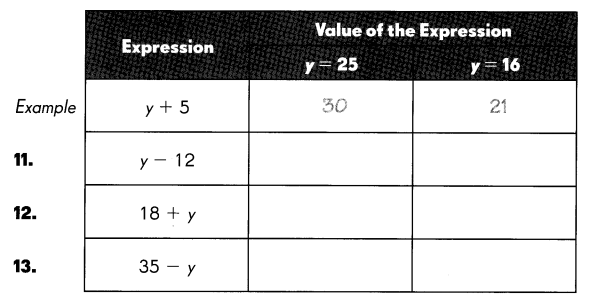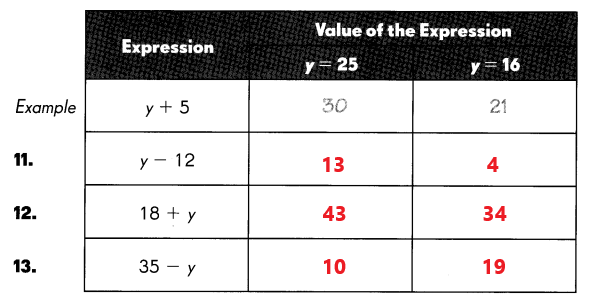Question 11.
y – 12
For y = 25
y – 12 = 25 – 12 = 13
For y = 16

y – 12 = 16 – 12 = 4
Explanation:
Place y = 25 in the expression y -12. Subtract 12 from 25 the difference is 13.
Place y = 16 in the expression y – 12. Subtract 12 from 16 the difference is 4.

Question 12.
18 + y
For y = 25
18 + y = 18 + 25 = 43
For y = 16

18 + y = 18 + 16 = 34
Explanation:
Place y = 25 in the expression 18 + y. Add 18 to 25 the sum is 43.
Place y = 16 in the expression 18 + y . Add 18 to 16  the sum is 34.

Question 13.
35 – y
For y = 25
35 – y = 35 – 25 = 10
For y = 16

35 – y = 35 – 16 = 19
Explanation:
Place y = 25 in the expression 35 – y. Subtract 25 from 35 the difference is 10.
Place y = 16 in the expression 35 – y. Subtract 16 from 35 the difference is 19.

Write each of the following in at least three other ways.

Example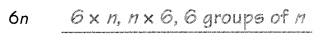Question 14.
18 × m _______
The three other ways of 6n is m × 18, 18 groups of n, 18n.

Question 15.
75 groups of y _______
The three other ways of 75 groups of y is 75y, 75 × y, y × 75.

Question 16.
y groups of 12 _______
The three other ways of  y groups of 12 is y12, y × 12, 12 × y.

Write an expression for each situation.

Question 17.
Julio has 4 boxes of pencils. There are 12 pencils in each box. How many pencils does Julio have?
4 × 12 = 48
Julio have 48 pencils.
Explanation:
Julio has 4 boxes of pencils. There are 12 pencils in each box. Multiply 4 with 12 the product is 48. Julio have 48 pencils.

Question 18.
Tara has k boxes of pencils. There are 10 pencils in each box. How many pencils does Tara have? Give your answer in terms of k.
k × 10 = 10k
Tara have 10k pencils.
Explanation:
Tara has k boxes of pencils. There are 10 pencils in each box. Multiply k with 10 the product is 10k. Tara have 10k pencils.

Write an expression for each situation.

Question 19.
A restaurant divided 20 gallons of lemonade among 4 containers. How much lemonade does each container contain?
20 ÷ 4 = 5
Explanation:
A restaurant divided 20 gallons of lemonade among 4 containers. Divide 20 by 4 the result is 5. Each container contains 5 lemonade.

Question 20.
m gallons of lemonade are distributed equally among 3 people. How much lemonade does each person get? Give your answer in terms of m.
m ÷ 3
Each person get m ÷ 3 lemonade.
Explanation:
m gallons of lemonade are distributed equally among 3 people. Divide m by 3. Each person get m ÷ 3 lemonade.

Question 21.
Multiply f and 6.
f × 6 = 6f

Question 22.
Divide m by 5.
m ÷ 5

Question 23.
Divide 22 by p.
22 ÷ p

Evaluate each expression for t = 156.

Example
2t = 2 × D
= 2 × 156
= 312

Question 24.
$$\frac{t}{6}$$ =
For t = 156
t/6 = 156 /6 = 26
Explanation:
Place t = 156 in given expression t/6. Divide 156 by 6 the result is 26.

Question 25.
16t =
For t = 156
16t = 16 × t = 16 × 156 = 2,496
Explanation:
Place t = 156 in given expression 6t. Multiply 16 with 156 the product is 2,496.

Question 26.
$$\frac{t}{13}$$ =
For t = 156
t/13= 156 /13 = 12
Explanation:
Place t = 156 in given expression t/13. Divide 156 by 13 the result is 12.

Write an algebraic expression for each situation.

Question 27.
A tank has x gallons of water. Ted adds 3 gallons of water into the tank. He pours the water equally into 4 smaller containers. How much water is in each container?

Question 28.
Jenny has $15. She buys 2 books that cost m dollars each. How much does she have left? Answer: She have (15 – 2m)$ left.
Jenny has $15. She buys 2 books that cost m dollars each. She have (15 – 2m)$ left.

Write an algebraic expression for each situation.

Question 29.
Betty collected 400 food packages for charity. She gave g packages to an orphanage, and distributed the rest equally among 4 charities. How many packages did each charity get?

Question 30.
To bake muffins, Matt needs x eggs for every 200 grams of flour. If he used 900 grams of flour, how many eggs did he use?

Write an expression for each situation.

Example
Subtract 12 from the product of 8 and a.
8 × a – 12 = 8a – 12

Question 31.
Add 14 to the product of 3 and b.
3 × b + 14 = 3b + 14
Explanation:
The expression for the situation add 14 to the product of 3 and b is 3b + 14.

Question 32.
Divide the product of 7 and d by 5.
7 × d ÷ 5 = 7d ÷ 5
Explanation:
The expression for the situation divide the product of 7 and d by 5 is 7d ÷ 5.

Evaluate each expression for x = 5.

Example
13x – 4 = 13 × 5 – 4
= 65 – 4
= 61

Question 33.
5x + 12 =
For x = 5
5x + 12 = 5 × 5 +12
= 25 + 12
= 37
Explanation:
Place x = 5 in the given expression 5x + 12. First multiply 5 with 5 the product is 25. Add 25 to 12 the sum is 37.

Question 34.
20 – 2x =
For x = 5
20 – 2x = 20 – 2 × 5
= 20 – 10
= 10
Explanation:
Place x = 5 in the given expression 20 – 2x. First multiply 2 with 5 the product is 10. Subtract 10 from the 20 the difference is 10.

Question 35.
$$\frac{x}{10}$$ + 2 =
For x = 5
x/10 + 2 = 5/10 + 2
= 1/2 + 2
= 0.5 + 2
2.5
Explanation:
Place x = 5 in the given expression x/10 + 2. First divide 5 by 10 the result is 1/2which is 0.5. Add 0.5 with 2 the sum is 2.5.

Question 36.
$$\frac{6 x}{5}$$ + 12 =
For x = 5
6x/5 + 12 = (6 × 5)/5 + 12
= 6 + 12
= 18
Explanation:
Place x = 5 in the given expression 6x/5 + 12. First multiply 6 with 5 the product is 30. Next divide 30 by 5 the result is 6. Add 6 with 12 the sum is 18.

Fill in the boxes with the correct expressions. In the last box on the right, evaluate each expression for m = 28.

Example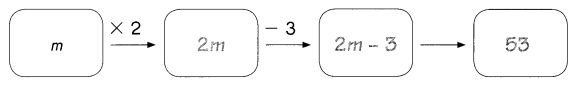Question 37.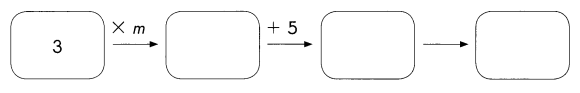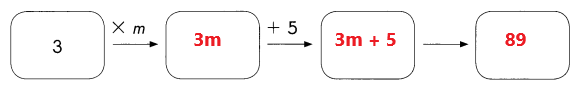For m= 28
3m + 5 = 3 × 28 + 5
= 84 + 5
= 89
Explanation:
In the above image we can observe four boxes. Multiply 3 with m the product is 3m. Next add 3m with 5 the sum is
3m + 5.
Place m = 28 in the expression 3m + 5. Multiply 3 with 28 the product is 84. Add 84 with 5 the sum is 89.

Question 38.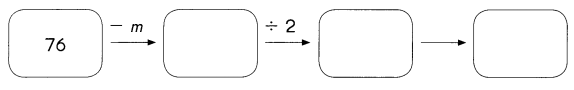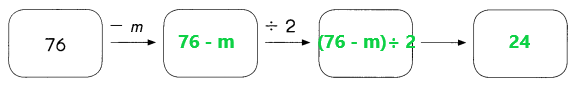For m = 28
(76 – m) ÷ 2 = (76 – 28) ÷ 2
= 48 ÷ 2
= 24
Explanation:
In the above image we can observe four boxes. Subtract m from 76. Next divide 76 – m by 2.
Place m = 28 in the expression (76 – m) ÷ 2. Subtract 28 from 76 the difference is 48. Divide 48 by 2 the result is 24.

Question 39.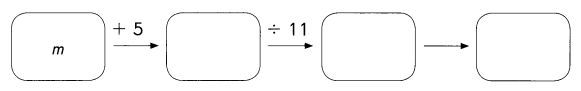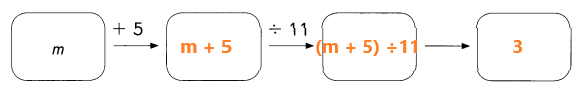For m = 28
(m + 5) ÷ 11 = (28 + 5) ÷ 11
= 33 ÷ 11
= 3
Explanation:
In the above image we can observe four boxes. Add m to 5. Next divide m + 5 by 11.
Place m = 28 in the expression (m + 5) ÷ 11. Add 28 with 5 the sum is 33. Divide 33 by 11 the result is 3.

Question 40.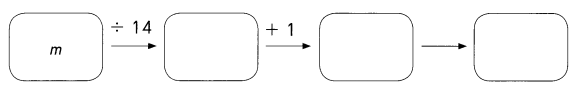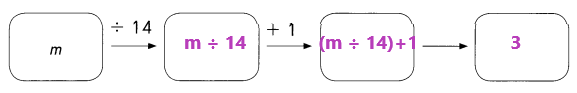For m = 28
(m ÷ 14) + 1 = (28 ÷ 14) + 1
= 2 + 1
= 3
Explanation:
In the above image we can observe four boxes. Divide m by 14. Next add (m ÷ 14) with 1.
Place m = 28 in the expression (m ÷ 14) + 1. Divide 28 by 14 the result is 2. Add 2 with 1 the sum is 3.

Question 41.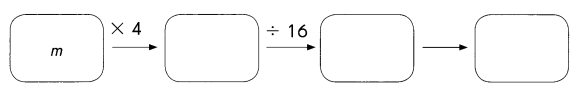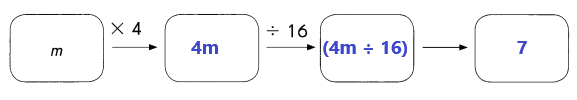For m = 28
4m ÷ 16 = 4 × 28 ÷ 16
= 112 ÷ 16
= 7
Explanation:
In the above image we can observe four boxes. Multiply m with 4 the product is 4m. Next divide 4m by 16 the result is 4m ÷ 16.
Place m = 28 in the expression 4m ÷ 16. Multiply 4 with 28 the product is 112. Divide 112 by 16 the result is 7.

Evaluate each expression for z = 1,256.

Question 42.
41 z – 39
For z = 1,256
41 z – 39 = 41 × 1,256 – 39
= 51,496 – 39
= 51,457
Explanation:
Place z = 1,256 in the given expression 41 z – 39. Multiply 41 with 1,256 the product is 51,496. Subtract 39 from 51,496 the difference is 51,457.

Question 43.
$$\frac{18,661-z}{5}$$
For z = 1,256
(18,661 – z) ÷  5 = (18,661 – 1,256) ÷  5
= 17,405 ÷  5
= 3,481
Explanation:
Place z = 1,256 in the given expression (18,661 – z) ÷  5. Subtract 1,256 from 18,661 the difference is 17,405. Divide 17,405 by 5 the result is 3,481.

Question 44.
$$\frac{13 z}{8}$$ + 7,389
For z = 1,256
(13 z ÷ 8) + 7,389 = (13 × 1,256 ÷ 8) + 7,389
= (16,328 ÷ 8) + 7,389
= 2,041 + 7,389
= 9,430
Explanation:
Place z = 1,256 in the given expression (13 z ÷ 8) + 7,389. Multiply 13 with 1,256 the product is 16,328. Divide 16,328 by 8 the result is 2,041. Add 2,041 with 7,389 the sum is 9,430.

Question 45.
$$\frac{9 z-1,476}{42}$$